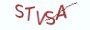Python基础教程

# 16.6.1 内置的dir方法

Python提供了一个内置函数dir，在dir函数中传递类型名或对象名，可以查看数据类型或对象中的属性和方法。dir的输出是一个列表，列表中包含的元素是数据类型中的属性和方法。现在进入Python交互模式，执行dir(dict)来查看字典中的所有属性和方法：
>>> dir(dict)
['__class__', '__contains__', '__delattr__', '__delitem__', '__dir__', '__doc__', '__eq__', '__format__', '__ge__', '__getattribute__', '__getitem__', '__gt__', '__hash__', '__init__', '__init_subclass__', '__iter__', '__le__', '__len__', '__lt__', '__ne__', '__new__', '__reduce__', '__reduce_ex__', '__repr__', '__setattr__', '__setitem__', '__sizeof__', '__str__', '__subclasshook__', 'clear', 'copy', 'fromkeys', 'get', 'items', 'keys', 'pop', 'popitem', 'setdefault', 'update', 'values']

# 16.6.2 常用的魔术属性

Python中常用的魔术属性主要有：__class__,__dict__,__doc__。

(1) __class__

```# 定义一个Cat类
class Cat:
def __init__(self, name):
self.__name = name

kitty = Cat("kitty")
print(kitty.__class__)

"""
(1) 执行print后的输出：
<class '__main__.Cat'>
(2) __class__的输出是一个类类型，所以可以通过__class__来进行对象的实例化

"""```
(2) __dict__:

```# __desc__ = 测试魔法属性__dict__

class Cat:
# 定义类属性species,来表示猫所属的物种
__species = "Cat"

@classmethod
def catch_mice(cls):
print("A cat that doesn't catch a mouse is not a good cat!")

def __init__(self，name):
# 在对象属性前面加上双下划线
self.__name = name

def miaow(self):
# 在类内部可以访问被"隐藏"的属性和方法
print("{} miaow when unhappy".format(self.__name))

# 访问类型中的__dict__
print(Cat.__dict__)
"""

{'__module__': '__main__', '_Cat__species': 'Cat', 'catch_mice': <classmethod object at 0x0000017C5EB6EE88>, '__init__': <function Cat.__init__ at 0x0000017C5EAFD5E8>, 'miaow': <function Cat.miaow at 0x0000017C5EAFD678>, '__dict__': <attribute '__dict__' of 'Cat' objects>, '__weakref__': <attribute '__weakref__' of 'Cat' objects>, '__doc__': None}
"""

# 访问对象中的__dict__
kitty = Cat("kitty")
print(kitty.__dict__)

"""

{'_Cat__name': 'kitty'}

"""```
（2） __doc__

__doc__表示文档字符串，文档字符串是一段是用三引号括住的字符串，出现在类体或函数体中的第一行，用来对类或函数进行功能及用法上的描述。
Python中的第三方工具会将文档字符串自动生成一个描述性的文档。

```# 定义一个Cat类
class Cat:
def __init__(self, name):
""" this cat is not a real cat,no rat """
self.__name = name

print(Cat.__doc__)

# 程序的输出为：this cat is not a real cat,no rat```

# 16.6.3 常用的魔术方法

(1) __str__

```# __desc__ = 重定义__str__方法

class Cat:
# 定义类属性species,来表示猫所属的物种
__species = "Cat"

def __init__(self，name):
# 在对象属性前面加上双下划线
self.__name = name

cat = Cat("kitty")
print(cat)

"""

<__main__.Cat object at 0x03738210>
"""

# 重定义__str__方法，修改对象的输出信息
class Cat:
# 定义类属性species,来表示猫所属的物种
__species = "Cat"

def __init__(self，name):
# 在对象属性前面加上双下划线
self.__name = name

def __str__(self):
# 该方法返回的是字符串类型
return "this cat is not a real cat,no rat"

cat = Cat("kitty")
print(cat)

"""

"this cat is not a real cat,no rat"
"""```
(2) __call__

```# __desc__ = 重定义__call__方法

class Cat:
# 定义类属性species,来表示猫所属的物种
__species = "Cat"

def __init__(self，name):
# 在对象属性前面加上双下划线
self.__name = name

def __call__(self):
return "this cat is not a real cat,no rat"

cat = Cat("kitty")
print(cat())

"""
(1)以函数调用的形式来调用对象
(2)Python会自动调用__call__方法，程序的输出为：
"this cat is not a real cat,no rat"
"""```
(3) __getattr__(self,name)

```# __desc__ = 通过__getattr__方法来实现惰性初始化

class Cat:
__species = "Cat"

def __init__(self, name):
self.__name = name
# 定义对象中的惰性属性名,键值为简单的lambda函数，用来模拟耗时的初始化
self.__lazy_attribute = {
"cry":lambda x:x*x
}

def __lazy_initialize(self, name):
return self.__lazy_attribute[name](0)

def __getattr__(self, name):
# 如果该属性为惰性属性，且还未初始化，那么现在进行初始化
if name in self.__lazy_attribute:
# __dict__保存了对象的属性，这样就相当于定义了name参数对应的属性
self.__dict__[name] = self.__lazy_initialize(name)
return self.__dict__[name]

return None

kitty = Cat("kitty")
# 访问不存在的cry属性,会执行__getattr__方法
print(kitty.cry)
# 程序的输出为0```

(4) __getitem__(self, key):

```# __desc__ = 定义__getitem__的方法，以操作符[]的形式对对象属性进行访问

class Cat:
__species = "Cat"

def __init__(self, name):
self.name = name

def __getitem__(self, name):
if name in self.__dict__:
# 如果为对象属性，就输出属性的值
return self.__dict__[name]

return None

cat = Cat("kitty")
"""

"""
print(cat["name"])
# 程序的输出为：kitty```

```# __desc__ = 通过__getitem__方法来实现迭代

class Cat:
__species = "Cat"
__cats = ["tiger", "lion"]

def __init__(self, name):
self.name = name

def __getitem__(self, index):
return self.__cats[index]

cats = Cat("kitty")
for cat in cats:
print(cat)

"""

tiger
lion
"""```
(5) __setitem__(self, key, value):

```# __desc__ = 通过__setitem__方法来实现[]操作

class Cat:
__species = "Cat"

def __init__(self, name):
self.name = name

def __setitem__(self, name, value):
self.__dict__[name] = value

kitty = Cat("kitty")

# 以[]的形式为属性赋值时会触发__setitem__方法的调用
kitty["price"] = 69

print(kitty.price)
# 程序的输出为：69```

# 16.6.4 最具实力的小班培训

`(1) Python后端工程师高薪就业班，月薪11K-18K，免费领取课程大纲(2) Python爬虫工程师高薪就业班，年薪十五万，免费领取课程大纲(3) Java后端开发工程师高薪就业班，月薪11K-20K, 免费领取课程大纲(4) Python大数据分析，量化投资就业班，月薪12K-25K,免费领取课程大纲`## 注册账号